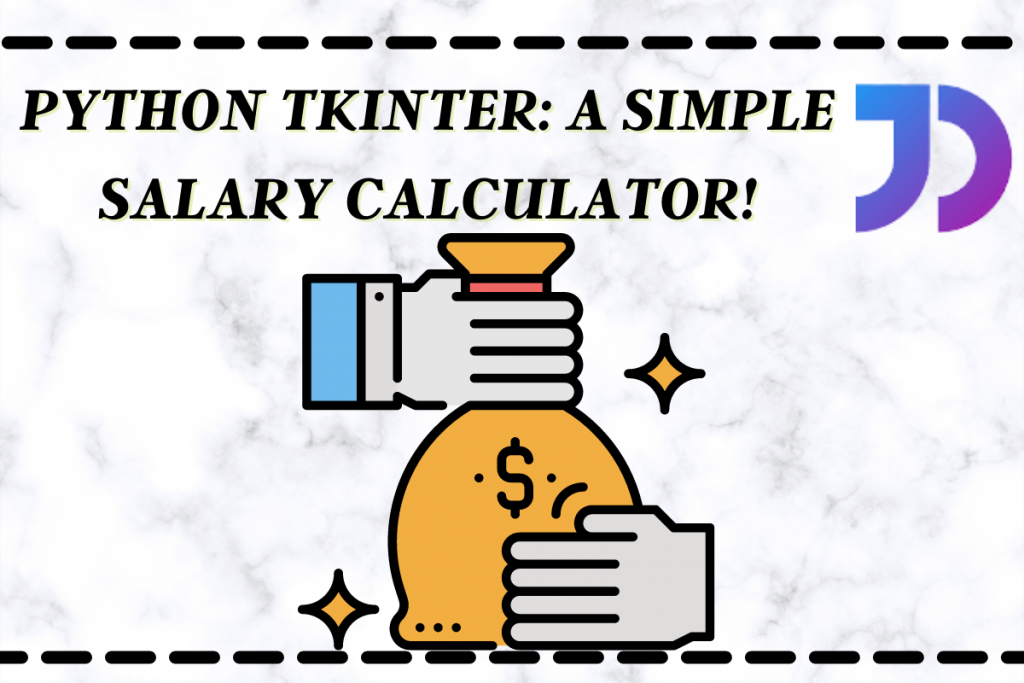# Python Tkinter: Simple Savings CalculatorHello learner! Today let’s use the Python Tkinter module to build a plain, GUI savings calculator.

The application takes on the salary of a person along with some expenditures such as travel, food and miscellaneous.The application will then determine the savings made my the person.

To create a application we will be doing the following things:

1. Importing the necessary library, in our case it’s `tkinter` library
2. Create the main window
3. Add any number of widgets to the main window (Button, Entry and Label)
4. Apply the event functionalities to the buttons

## Designing the Savings Calculator in Python

The designing of the application includes creating and configuring a the main window. It also involves adding all the necessary widgets on the screen.

The code for the same is shown below.

```import tkinter as tk
window = tk.Tk()
window.geometry("300x400")
window.config(bg="#F39C12")
window.resizable(width=False,height=False)
window.title('Savings Calculator')

l1 = tk.Label(window,text="Enter the Values",font=("Arial",20),fg="Black",bg="White")

l2 = tk.Label(window,text="Total Salary:",font=("Arial",10),fg="Black",bg="White")
e1 = tk.Entry(window,font=("Arial",11))

l3 = tk.Label(window,text="Travel:",font=("Arial",10),fg="Black",bg="White")
e2 = tk.Entry(window,font=("Arial",11))

l4 = tk.Label(window,text="Food:",font=("Arial",10),fg="Black",bg="White")
e3 = tk.Entry(window,font=("Arial",11))

l5 = tk.Label(window,text="Miscellaneous:",font=("Arial",10),fg="Black",bg="White")
e4 = tk.Entry(window,font=("Arial",11))

b1 = tk.Button(window,text="Calculate Savings",font=("Arial",15))

e5 = tk.Entry(window,font=("Arial",11),state='disabled')

b2 = tk.Button(window,text="Clear Values",font=("Arial",15))
b3 = tk.Button(window,text="Exit Application",font=("Arial",15))

l1.place(x=50,y=20)
l2.place(x=20,y=70)
e1.place(x=120,y=70)
l3.place(x=20,y=100)
e2.place(x=120,y=100)
l4.place(x=20,y=130)
e3.place(x=120,y=130)
l5.place(x=20,y=160)
e4.place(x=120,y=160)
b1.place(x=60,y=200)
l6.place(x=20,y=260)
e5.place(x=120,y=260)
b2.place(x=70,y=300)
b3.place(x=60,y=350)

window.mainloop()
```

If you have any issue or doubt about any widget we have used you can refer to the tutorial here. The final design of the application is shown below.

## Adding Functionality to the application

In order to add the functionalities we need to take both `entry` and `button` widgets into consideration.

### Working of Entry Boxes

For each entry box, we create variables that stores the value of the entry box. To create a variable to store integer values we make use of `StringVar` function.

After assigining a unique variable for each entry box we add a `textvariable` attribute to the entry box declaration to connect the variable with the entry box.

The code for the same is shown below. The changes made in the code have been highlighted for you.

```import tkinter as tk
window = tk.Tk()
window.geometry("300x400")
window.config(bg="#F39C12")
window.resizable(width=False,height=False)
window.title('Savings Calculator')

v1 = tk.StringVar()
v2 = tk.StringVar()
v3 = tk.StringVar()
v4 = tk.StringVar()
v5 = tk.StringVar()

l1 = tk.Label(window,text="Enter the Values",font=("Arial",20),fg="Black",bg="White")

l2 = tk.Label(window,text="Total Salary:",font=("Arial",10),fg="Black",bg="White")
e1 = tk.Entry(window,font=("Arial",11),textvariable=v1)

l3 = tk.Label(window,text="Travel:",font=("Arial",10),fg="Black",bg="White")
e2 = tk.Entry(window,font=("Arial",11),textvariable=v2)

l4 = tk.Label(window,text="Food:",font=("Arial",10),fg="Black",bg="White")
e3 = tk.Entry(window,font=("Arial",11),textvariable=v3)

l5 = tk.Label(window,text="Miscellaneous:",font=("Arial",10),fg="Black",bg="White")
e4 = tk.Entry(window,font=("Arial",11),textvariable=v4)

b1 = tk.Button(window,text="Calculate Savings",font=("Arial",15))

e5 = tk.Entry(window,font=("Arial",11),state='disabled',textvariable=v5)

b2 = tk.Button(window,text="Clear Values",font=("Arial",15))
b3 = tk.Button(window,text="Exit Application",font=("Arial",15))

l1.place(x=50,y=20)
l2.place(x=20,y=70)
e1.place(x=120,y=70)
l3.place(x=20,y=100)
e2.place(x=120,y=100)
l4.place(x=20,y=130)
e3.place(x=120,y=130)
l5.place(x=20,y=160)
e4.place(x=120,y=160)
b1.place(x=60,y=200)
l6.place(x=20,y=260)
e5.place(x=120,y=260)
b2.place(x=70,y=300)
b3.place(x=60,y=350)

window.mainloop()
```

### Working of Buttons

In our application, we have three buttons. Hence, we will be defining three different functions for the buttons. The codes for all three functions are defined below.

```def exit():
window.destroy()

def clear_all():
e1.delete(0,tk.END)
e2.delete(0,tk.END)
e3.delete(0,tk.END)
e4.delete(0,tk.END)
e5.config(state='normal')
e5.delete(0,tk.END)
e5.config(state='disabled')

def cal_savings():
e5.config(state='normal')
e5.delete(0,tk.END)
e5.config(state='disabled')
salary = int(e1.get())
total_expenditure = int(e2.get())+int(e3.get())+int(e4.get())
savings = salary - total_expenditure
e5.config(state='normal')
e5.insert(0,savings)
e5.config(state='disabled')
```

For the first function we just destroy the window we created. And for the second funtion we delete contents of all the entry boxes.

For the third function, we add up all the expenditure values and take the difference between the total salary and the expenditure. But before this, we will clear the output entry box to make space for the new values.

We then put the calculated value inside the output entry box. After the functions are created we add the `command` parameter into the button declarations.

There you go! You are all set!

## Implementing a Savings Calculator in Tkinter

The complete code for the application is given below.

```def exit():
window.destroy()

def clear_all():
e1.delete(0,tk.END)
e2.delete(0,tk.END)
e3.delete(0,tk.END)
e4.delete(0,tk.END)
e5.config(state='normal')
e5.delete(0,tk.END)
e5.config(state='disabled')

def cal_savings():
e5.config(state='normal')
e5.delete(0,tk.END)
e5.config(state='disabled')
salary = int(e1.get())
total_expenditure = int(e2.get())+int(e3.get())+int(e4.get())
savings = salary - total_expenditure
e5.config(state='normal')
e5.insert(0,savings)
e5.config(state='disabled')

import tkinter as tk
window = tk.Tk()
window.geometry("300x400")
window.config(bg="#F39C12")
window.resizable(width=False,height=False)
window.title('Savings Calculator')

v1 = tk.StringVar()
v2 = tk.StringVar()
v3 = tk.StringVar()
v4 = tk.StringVar()
v5 = tk.StringVar()

l1 = tk.Label(window,text="Enter the Values",font=("Arial",20),fg="Black",bg="White")

l2 = tk.Label(window,text="Total Salary:",font=("Arial",10),fg="Black",bg="White")
e1 = tk.Entry(window,font=("Arial",11),textvariable=v1)

l3 = tk.Label(window,text="Travel:",font=("Arial",10),fg="Black",bg="White")
e2 = tk.Entry(window,font=("Arial",11),textvariable=v2)

l4 = tk.Label(window,text="Food:",font=("Arial",10),fg="Black",bg="White")
e3 = tk.Entry(window,font=("Arial",11),textvariable=v3)

l5 = tk.Label(window,text="Miscellaneous:",font=("Arial",10),fg="Black",bg="White")
e4 = tk.Entry(window,font=("Arial",11),textvariable=v4)

b1 = tk.Button(window,text="Calculate Savings",font=("Arial",15),command=cal_savings)

e5 = tk.Entry(window,font=("Arial",11),state='disabled',textvariable=v5)

b2 = tk.Button(window,text="Clear Values",font=("Arial",15),command=clear_all)
b3 = tk.Button(window,text="Exit Application",font=("Arial",15),command=exit)

l1.place(x=50,y=20)
l2.place(x=20,y=70)
e1.place(x=120,y=70)
l3.place(x=20,y=100)
e2.place(x=120,y=100)
l4.place(x=20,y=130)
e3.place(x=120,y=130)
l5.place(x=20,y=160)
e4.place(x=120,y=160)
b1.place(x=60,y=200)
l6.place(x=20,y=260)
e5.place(x=120,y=260)
b2.place(x=70,y=300)
b3.place(x=60,y=350)

window.mainloop()
```

Output: The application was tested for a variety of numerical values. A few of them are shown below.